FreeRDP
tsmf_media.c File Reference
`#include <stdio.h>`
`#include <stdlib.h>`
`#include <string.h>`
`#include <signal.h>`
`#include <sys/time.h>`
`#include <winpr/crt.h>`
`#include <winpr/synch.h>`
`#include <winpr/thread.h>`
`#include <winpr/stream.h>`
`#include <winpr/collections.h>`
`#include <freerdp/client/tsmf.h>`
`#include "tsmf_constants.h"`
`#include "tsmf_types.h"`
`#include "tsmf_decoder.h"`
`#include "tsmf_audio.h"`
`#include "tsmf_main.h"`
`#include "tsmf_codec.h"`
`#include "tsmf_media.h"`

## Macros

#define AUDIO_TOLERANCE   10000000LL

#define VIDEO_ADJUST_MAX   10 * 1000 * 1000

#define MAX_ACK_TIME   666667

#define AUDIO_MIN_BUFFER_LEVEL   3

#define AUDIO_MAX_BUFFER_LEVEL   6

#define VIDEO_MIN_BUFFER_LEVEL   10

#define VIDEO_MAX_BUFFER_LEVEL   30

## Functions

static void _tsmf_presentation_free (void *obj)

static void _tsmf_stream_free (void *obj)

static UINT64 get_current_time (void)

static TSMF_SAMPLE * tsmf_stream_pop_sample (TSMF_STREAM *stream, int sync)

static void tsmf_sample_free (void *arg)

static BOOL tsmf_sample_ack (TSMF_SAMPLE *sample)

static BOOL tsmf_sample_queue_ack (TSMF_SAMPLE *sample)

static BOOL tsmf_stream_process_ack (void *arg, BOOL force)

TSMF_PRESENTATION * tsmf_presentation_new (const BYTE *guid, IWTSVirtualChannelCallback *pChannelCallback)

static char * guid_to_string (const BYTE *guid, char *str, size_t len)

TSMF_PRESENTATION * tsmf_presentation_find_by_id (const BYTE *guid)

static BOOL tsmf_sample_playback_video (TSMF_SAMPLE *sample)

static BOOL tsmf_sample_playback_audio (TSMF_SAMPLE *sample)

static BOOL tsmf_sample_playback (TSMF_SAMPLE *sample)

static DWORD WINAPI tsmf_stream_ack_func (LPVOID arg)

static DWORD WINAPI tsmf_stream_playback_func (LPVOID arg)

static BOOL tsmf_stream_start (TSMF_STREAM *stream)

static BOOL tsmf_stream_stop (TSMF_STREAM *stream)

static BOOL tsmf_stream_pause (TSMF_STREAM *stream)

static BOOL tsmf_stream_restart (TSMF_STREAM *stream)

static BOOL tsmf_stream_change_volume (TSMF_STREAM *stream, UINT32 newVolume, UINT32 muted)

BOOL tsmf_presentation_volume_changed (TSMF_PRESENTATION *presentation, UINT32 newVolume, UINT32 muted)

BOOL tsmf_presentation_paused (TSMF_PRESENTATION *presentation)

BOOL tsmf_presentation_restarted (TSMF_PRESENTATION *presentation)

BOOL tsmf_presentation_start (TSMF_PRESENTATION *presentation)

UINT tsmf_presentation_sync (TSMF_PRESENTATION *presentation)

BOOL tsmf_presentation_stop (TSMF_PRESENTATION *presentation)

BOOL tsmf_presentation_set_geometry_info (TSMF_PRESENTATION *presentation, UINT32 x, UINT32 y, UINT32 width, UINT32 height, int num_rects, RDP_RECT *rects)

void tsmf_presentation_set_audio_device (TSMF_PRESENTATION *presentation, const char *name, const char *device)

BOOL tsmf_stream_flush (TSMF_STREAM *stream)

void tsmf_presentation_free (TSMF_PRESENTATION *presentation)

TSMF_STREAM * tsmf_stream_new (TSMF_PRESENTATION *presentation, UINT32 stream_id, rdpContext *rdpcontext)

TSMF_STREAM * tsmf_stream_find_by_id (TSMF_PRESENTATION *presentation, UINT32 stream_id)

static void tsmf_stream_resync (void *arg)

BOOL tsmf_stream_set_format (TSMF_STREAM *stream, const char *name, wStream *s)

void tsmf_stream_end (TSMF_STREAM *stream, UINT32 message_id, IWTSVirtualChannelCallback *pChannelCallback)

void tsmf_stream_free (TSMF_STREAM *stream)

BOOL tsmf_stream_push_sample (TSMF_STREAM *stream, IWTSVirtualChannelCallback *pChannelCallback, UINT32 sample_id, UINT64 start_time, UINT64 end_time, UINT64 duration, UINT32 extensions, UINT32 data_size, BYTE *data)

static void tsmf_signal_handler (int s)

BOOL tsmf_media_init (void)

## Variables

static wArrayList * presentation_list = NULL

static int TERMINATING = 0

## ◆ AUDIO_MAX_BUFFER_LEVEL

 #define AUDIO_MAX_BUFFER_LEVEL   6

## ◆ AUDIO_MIN_BUFFER_LEVEL

 #define AUDIO_MIN_BUFFER_LEVEL   3

## ◆ AUDIO_TOLERANCE

 #define AUDIO_TOLERANCE   10000000LL

FreeRDP: A Remote Desktop Protocol Implementation Video Redirection Virtual Channel - Media Container

Licensed under the Apache License, Version 2.0 (the "License"); you may not use this file except in compliance with the License. You may obtain a copy of the License at

```http://www.apache.org/licenses/LICENSE-2.0
```

Unless required by applicable law or agreed to in writing, software distributed under the License is distributed on an "AS IS" BASIS, WITHOUT WARRANTIES OR CONDITIONS OF ANY KIND, either express or implied. See the License for the specific language governing permissions and limitations under the License.

## ◆ MAX_ACK_TIME

 #define MAX_ACK_TIME   666667

 #define VIDEO_ADJUST_MAX   10 * 1000 * 1000

## ◆ VIDEO_MAX_BUFFER_LEVEL

 #define VIDEO_MAX_BUFFER_LEVEL   30

## ◆ VIDEO_MIN_BUFFER_LEVEL

 #define VIDEO_MIN_BUFFER_LEVEL   10

## ◆ _tsmf_presentation_free()

 void _tsmf_presentation_free ( void * obj )
static
Here is the call graph for this function: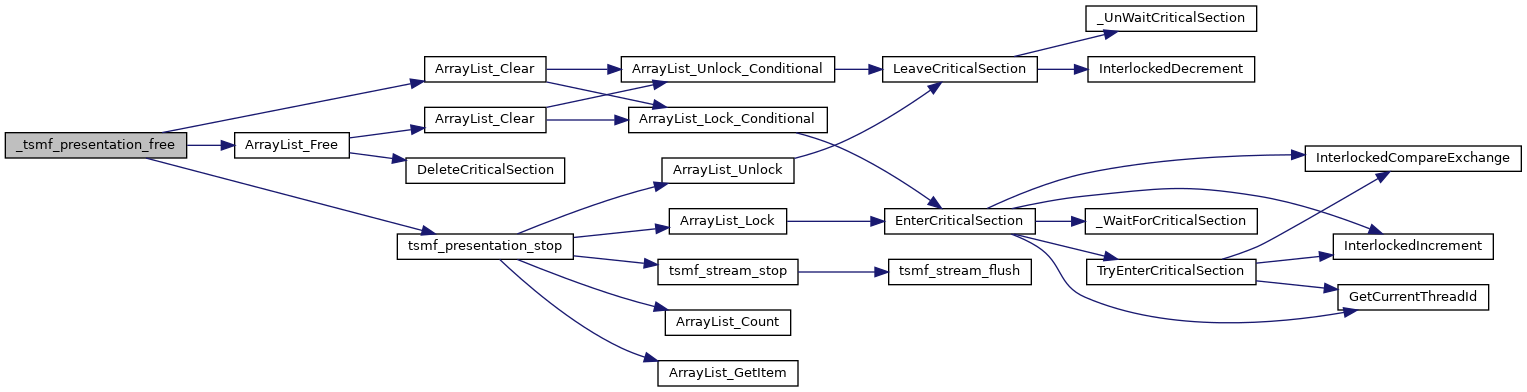Here is the caller graph for this function: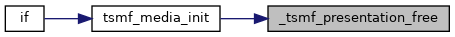## ◆ _tsmf_stream_free()

 void _tsmf_stream_free ( void * obj )
static
Here is the call graph for this function:Here is the caller graph for this function: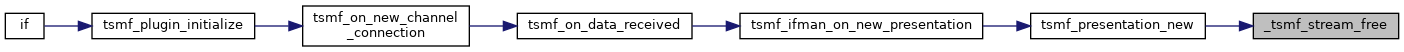## ◆ get_current_time()

 static UINT64 get_current_time ( void )
static
Here is the caller graph for this function:## ◆ guid_to_string()

 static char* guid_to_string ( const BYTE * guid, char * str, size_t len )
static
Here is the caller graph for this function: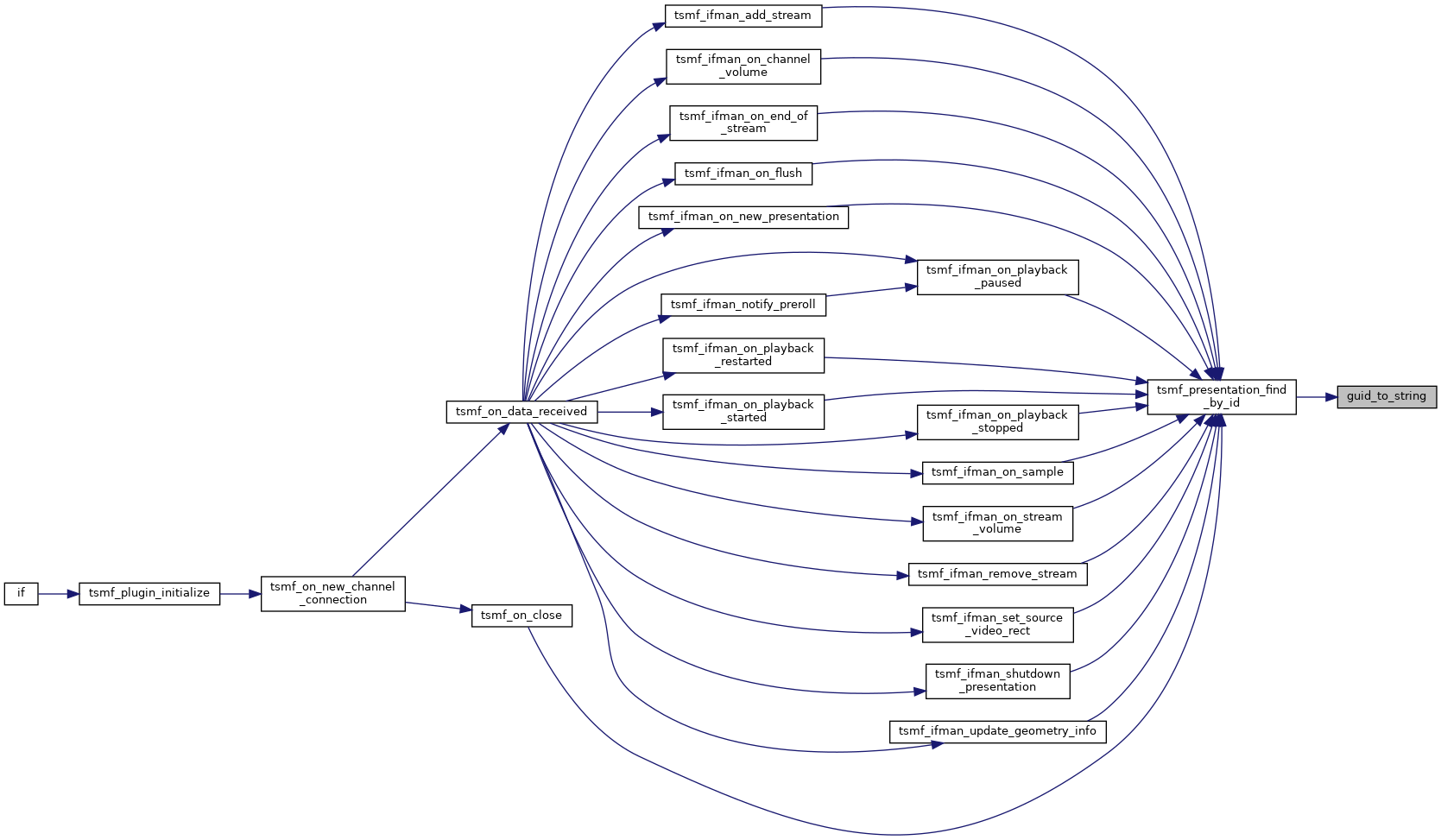## ◆ tsmf_media_init()

 BOOL tsmf_media_init ( void )
Here is the call graph for this function: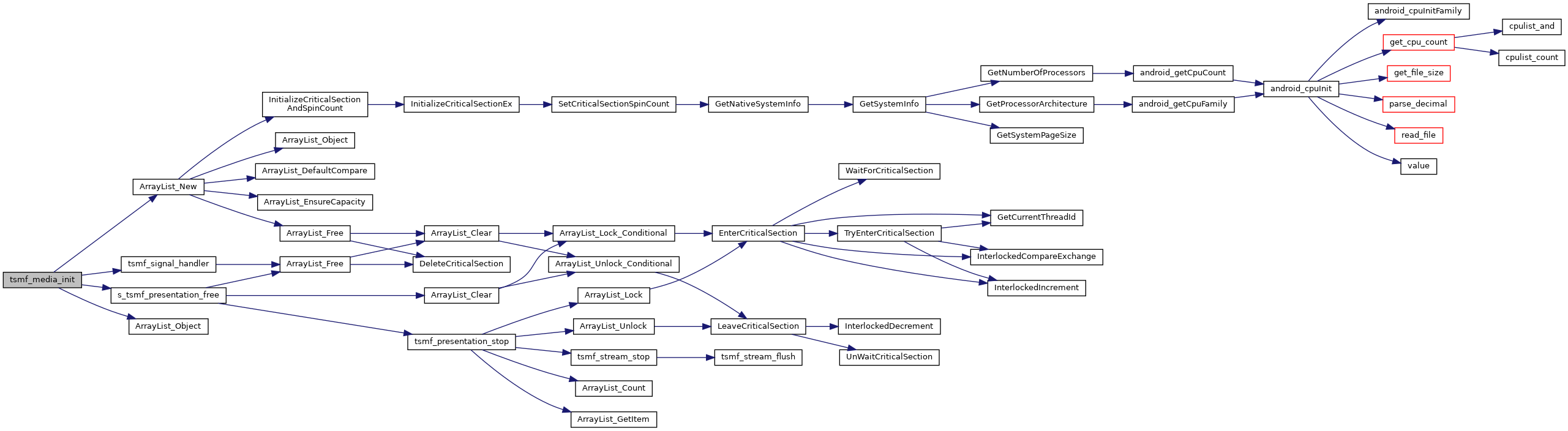Here is the caller graph for this function: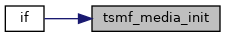## ◆ tsmf_presentation_find_by_id()

 TSMF_PRESENTATION* tsmf_presentation_find_by_id ( const BYTE * guid )
Here is the call graph for this function: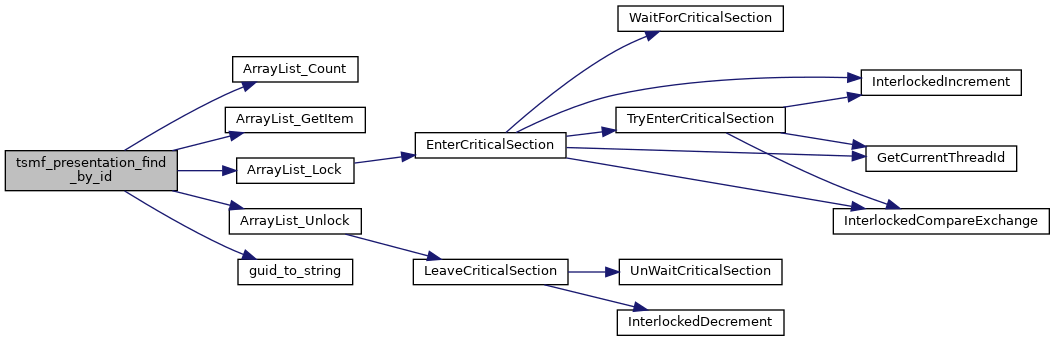Here is the caller graph for this function: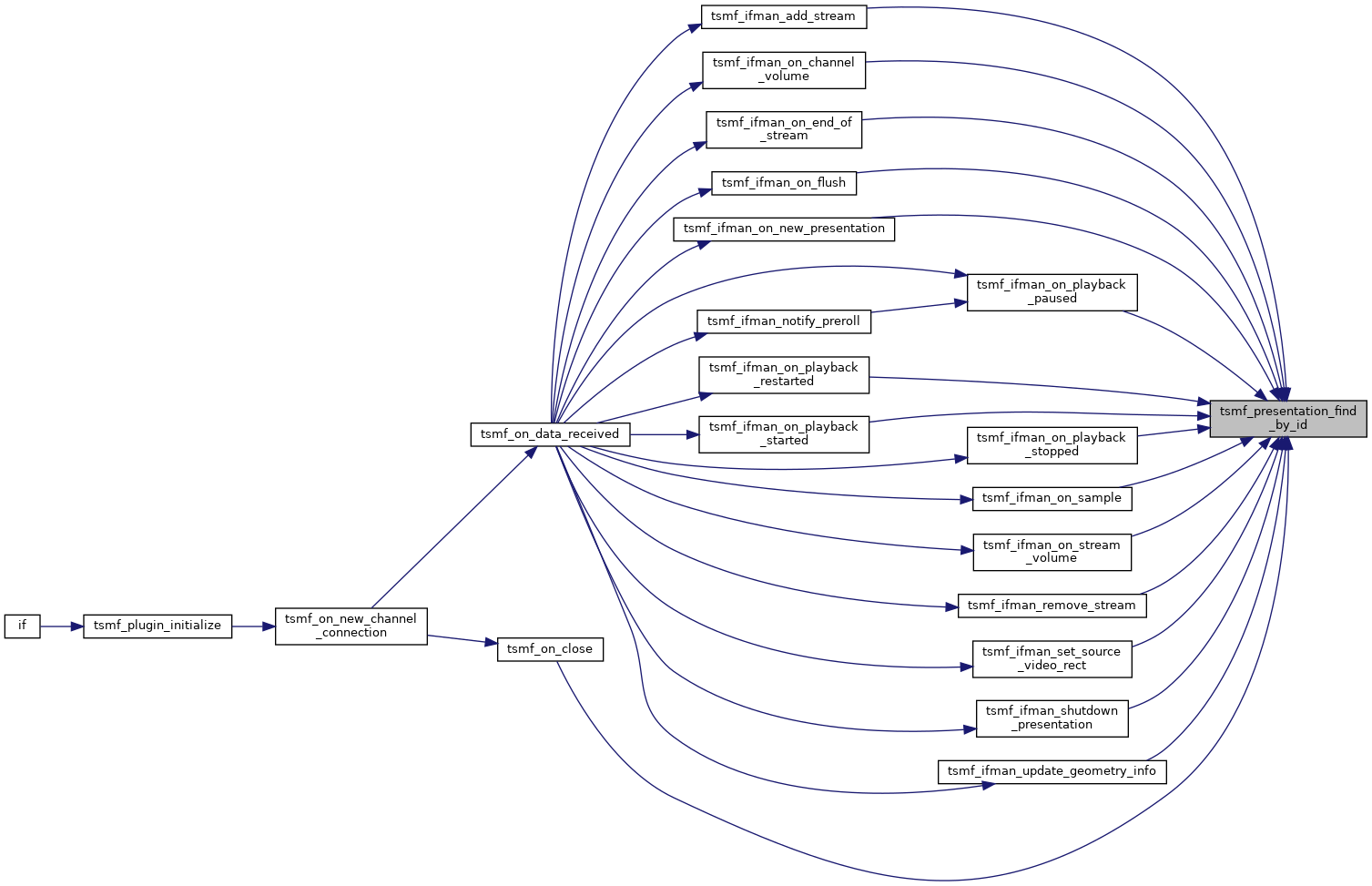## ◆ tsmf_presentation_free()

 void tsmf_presentation_free ( TSMF_PRESENTATION * presentation )
Here is the call graph for this function: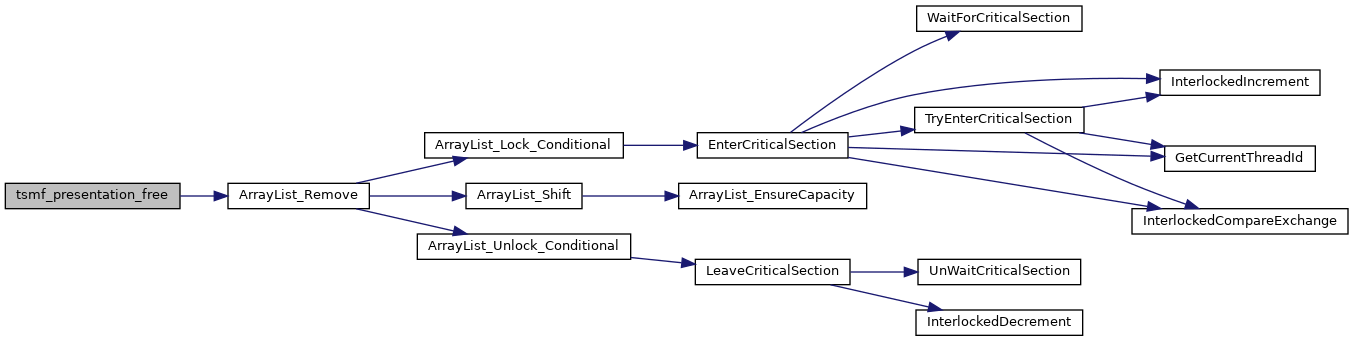Here is the caller graph for this function: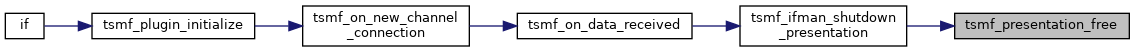## ◆ tsmf_presentation_new()

 TSMF_PRESENTATION* tsmf_presentation_new ( const BYTE * guid, IWTSVirtualChannelCallback * pChannelCallback )
Here is the call graph for this function: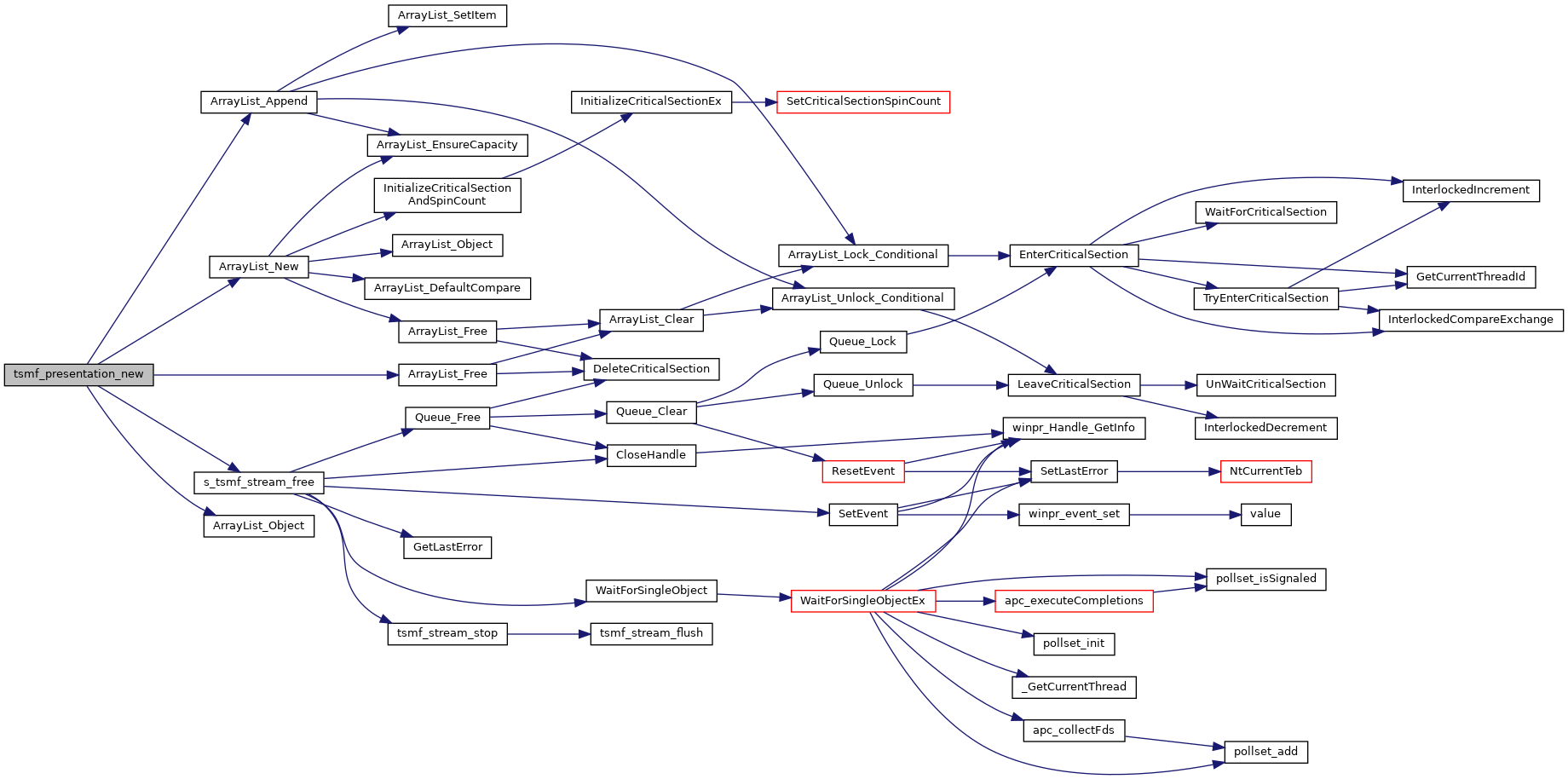Here is the caller graph for this function:## ◆ tsmf_presentation_paused()

 BOOL tsmf_presentation_paused ( TSMF_PRESENTATION * presentation )
Here is the call graph for this function: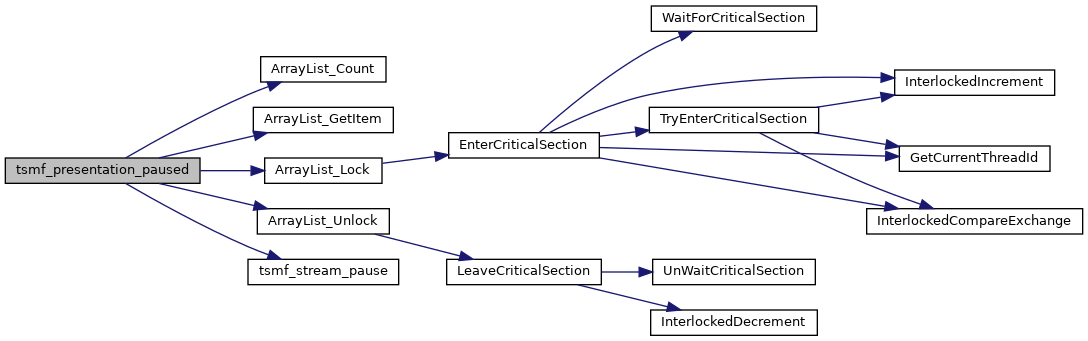Here is the caller graph for this function:## ◆ tsmf_presentation_restarted()

 BOOL tsmf_presentation_restarted ( TSMF_PRESENTATION * presentation )
Here is the call graph for this function: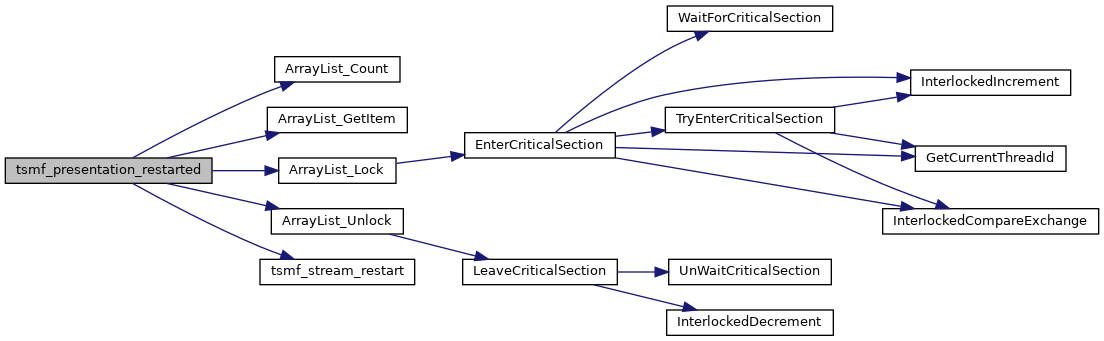Here is the caller graph for this function: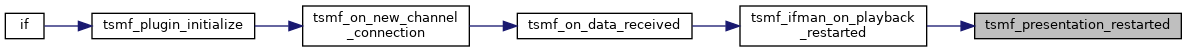## ◆ tsmf_presentation_set_audio_device()

 void tsmf_presentation_set_audio_device ( TSMF_PRESENTATION * presentation, const char * name, const char * device )
Here is the caller graph for this function:## ◆ tsmf_presentation_set_geometry_info()

 BOOL tsmf_presentation_set_geometry_info ( TSMF_PRESENTATION * presentation, UINT32 x, UINT32 y, UINT32 width, UINT32 height, int num_rects, RDP_RECT * rects )
Here is the call graph for this function:Here is the caller graph for this function: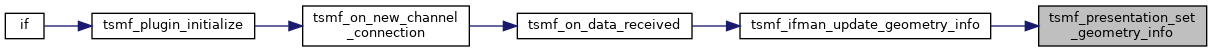## ◆ tsmf_presentation_start()

 BOOL tsmf_presentation_start ( TSMF_PRESENTATION * presentation )
Here is the call graph for this function: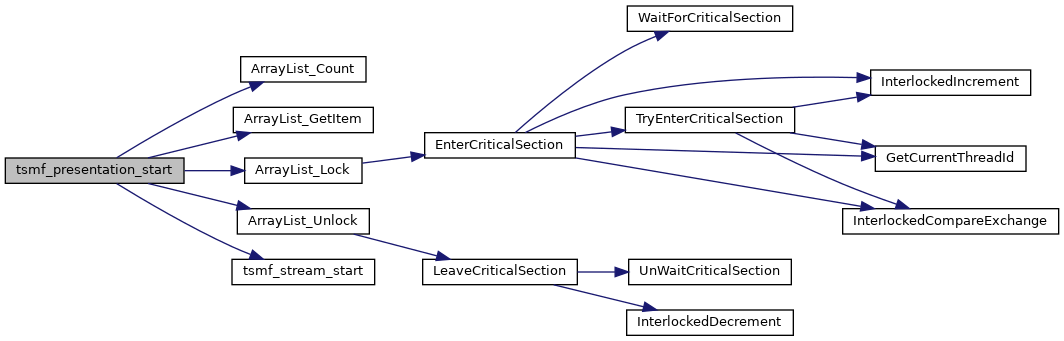Here is the caller graph for this function: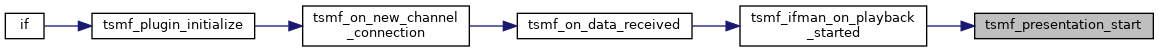## ◆ tsmf_presentation_stop()

 BOOL tsmf_presentation_stop ( TSMF_PRESENTATION * presentation )
Here is the call graph for this function:Here is the caller graph for this function: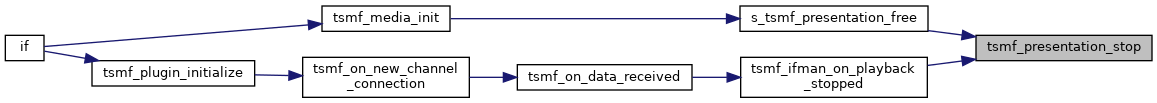## ◆ tsmf_presentation_sync()

 UINT tsmf_presentation_sync ( TSMF_PRESENTATION * presentation )

Function description

Returns
0 on success, otherwise a Win32 error code
Here is the call graph for this function: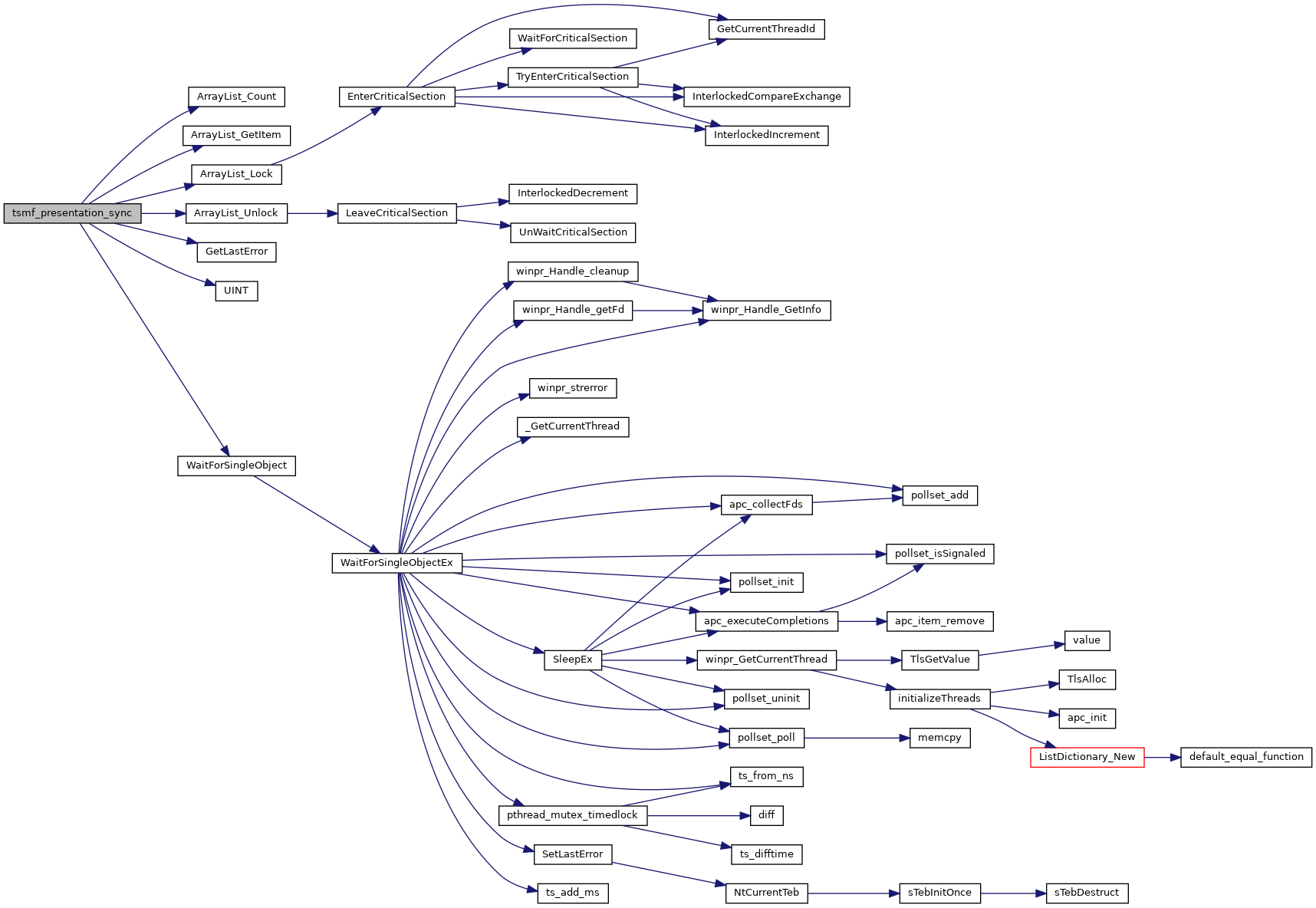Here is the caller graph for this function: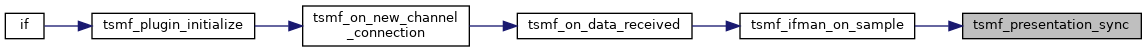## ◆ tsmf_presentation_volume_changed()

 BOOL tsmf_presentation_volume_changed ( TSMF_PRESENTATION * presentation, UINT32 newVolume, UINT32 muted )
Here is the call graph for this function: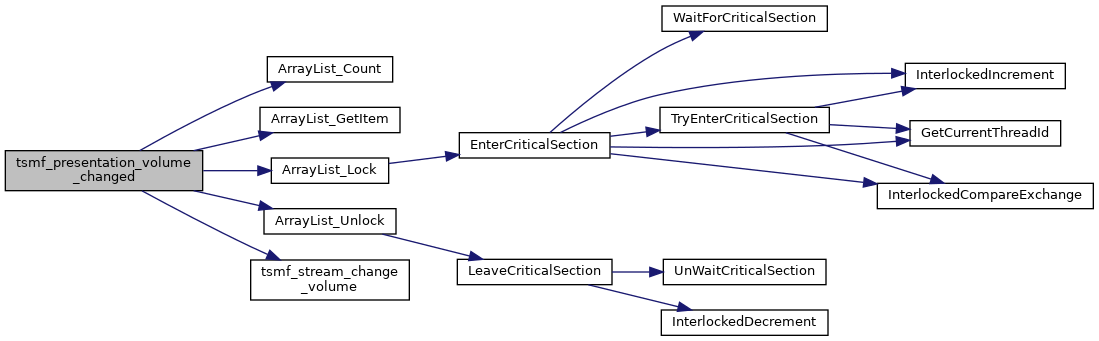Here is the caller graph for this function:## ◆ tsmf_sample_ack()

 static BOOL tsmf_sample_ack ( TSMF_SAMPLE * sample )
static
Here is the call graph for this function: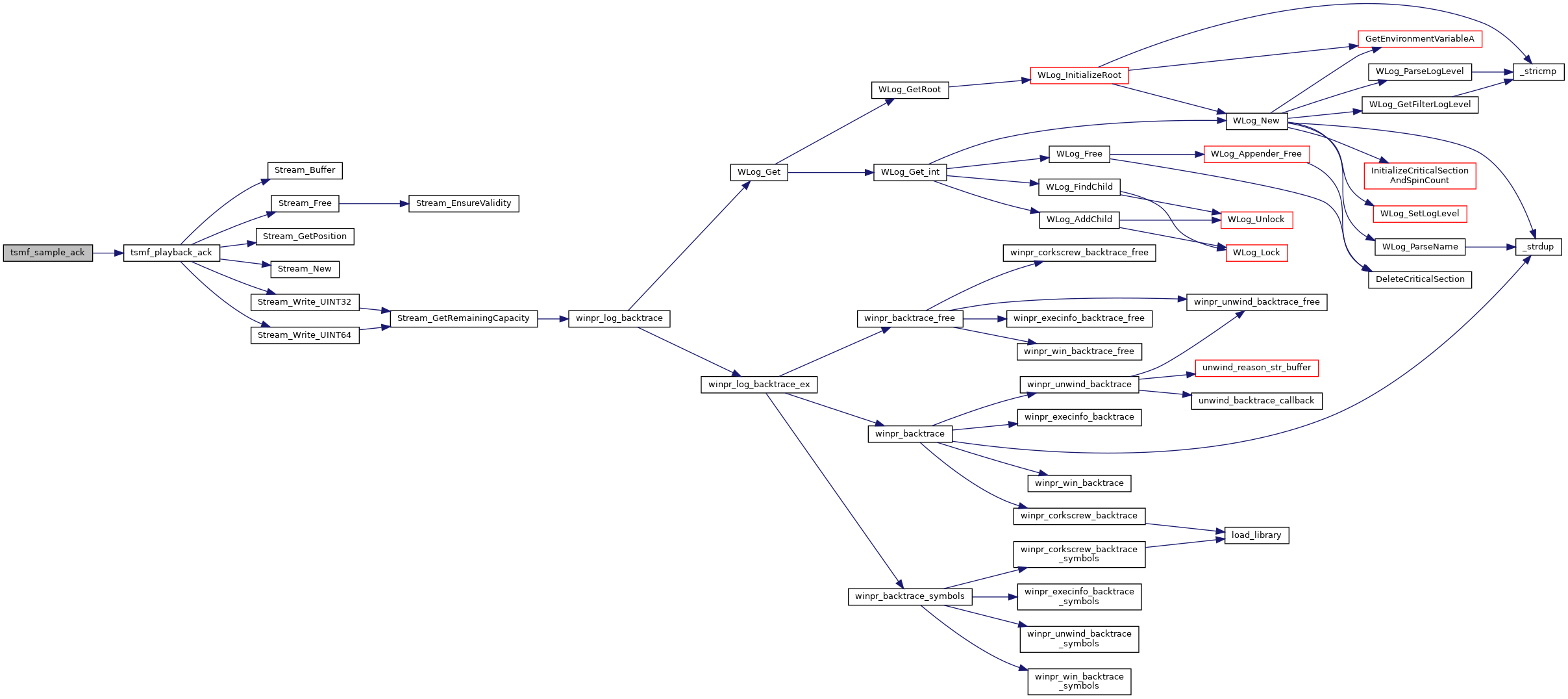Here is the caller graph for this function: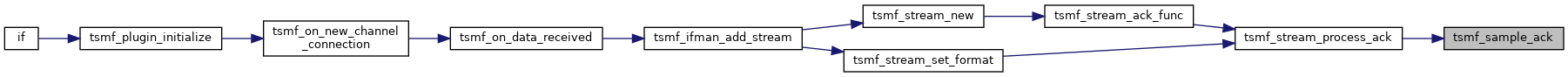## ◆ tsmf_sample_free()

 static void tsmf_sample_free ( void * arg )
static
Here is the caller graph for this function: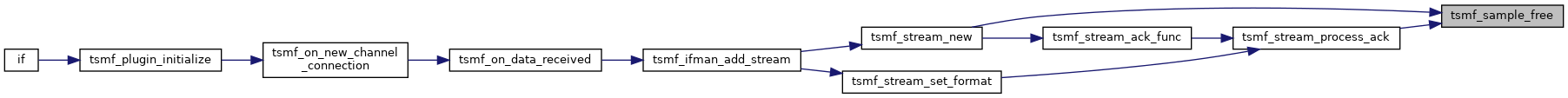## ◆ tsmf_sample_playback()

 static BOOL tsmf_sample_playback ( TSMF_SAMPLE * sample )
static
Here is the call graph for this function: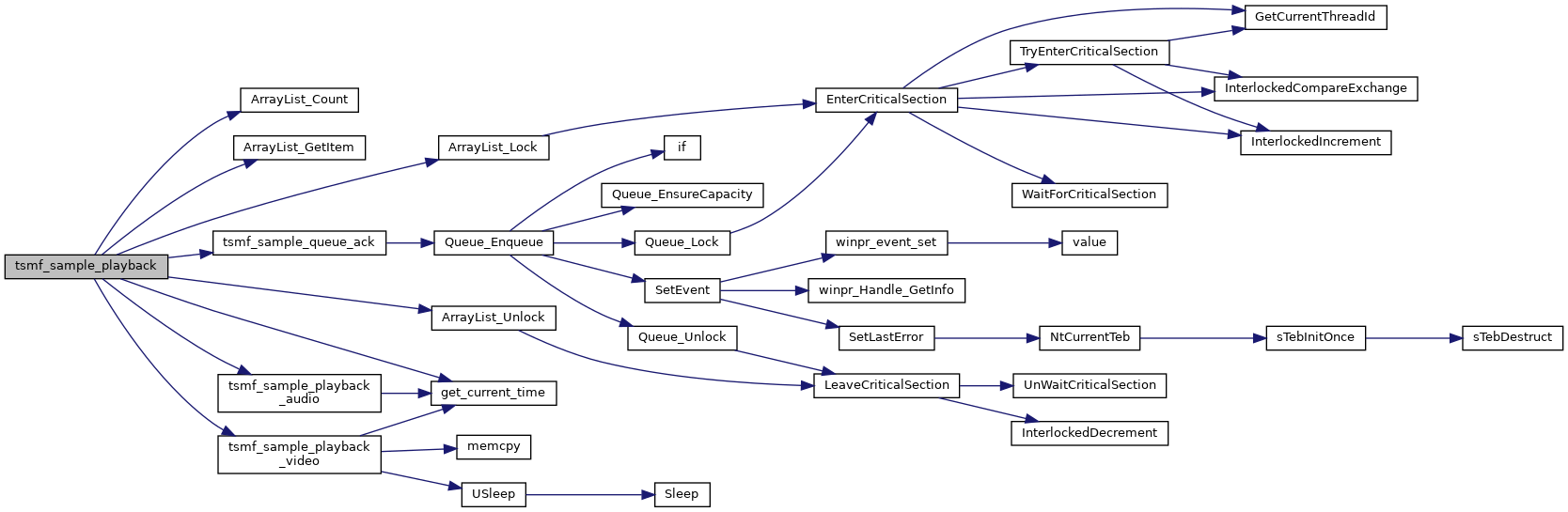Here is the caller graph for this function: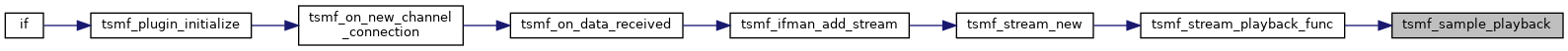## ◆ tsmf_sample_playback_audio()

 static BOOL tsmf_sample_playback_audio ( TSMF_SAMPLE * sample )
static
Here is the call graph for this function: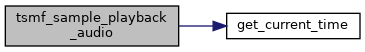Here is the caller graph for this function: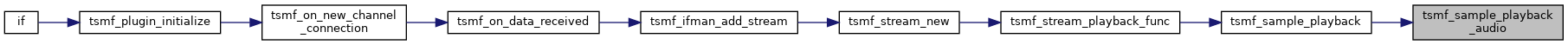## ◆ tsmf_sample_playback_video()

 static BOOL tsmf_sample_playback_video ( TSMF_SAMPLE * sample )
static
Here is the call graph for this function: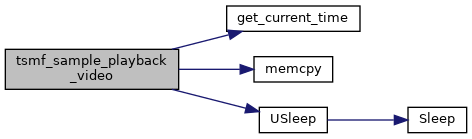Here is the caller graph for this function: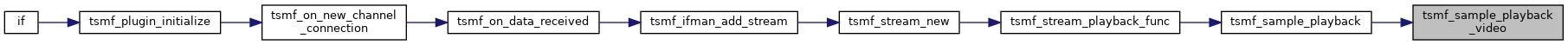## ◆ tsmf_sample_queue_ack()

 static BOOL tsmf_sample_queue_ack ( TSMF_SAMPLE * sample )
static
Here is the call graph for this function:Here is the caller graph for this function: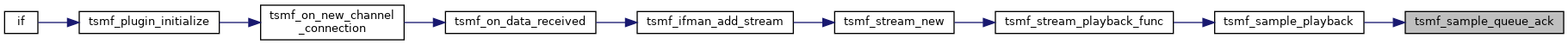## ◆ tsmf_signal_handler()

 static void tsmf_signal_handler ( int s )
static
Here is the call graph for this function:Here is the caller graph for this function: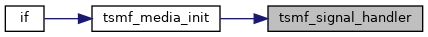## ◆ tsmf_stream_ack_func()

 static DWORD WINAPI tsmf_stream_ack_func ( LPVOID arg )
static
Here is the call graph for this function: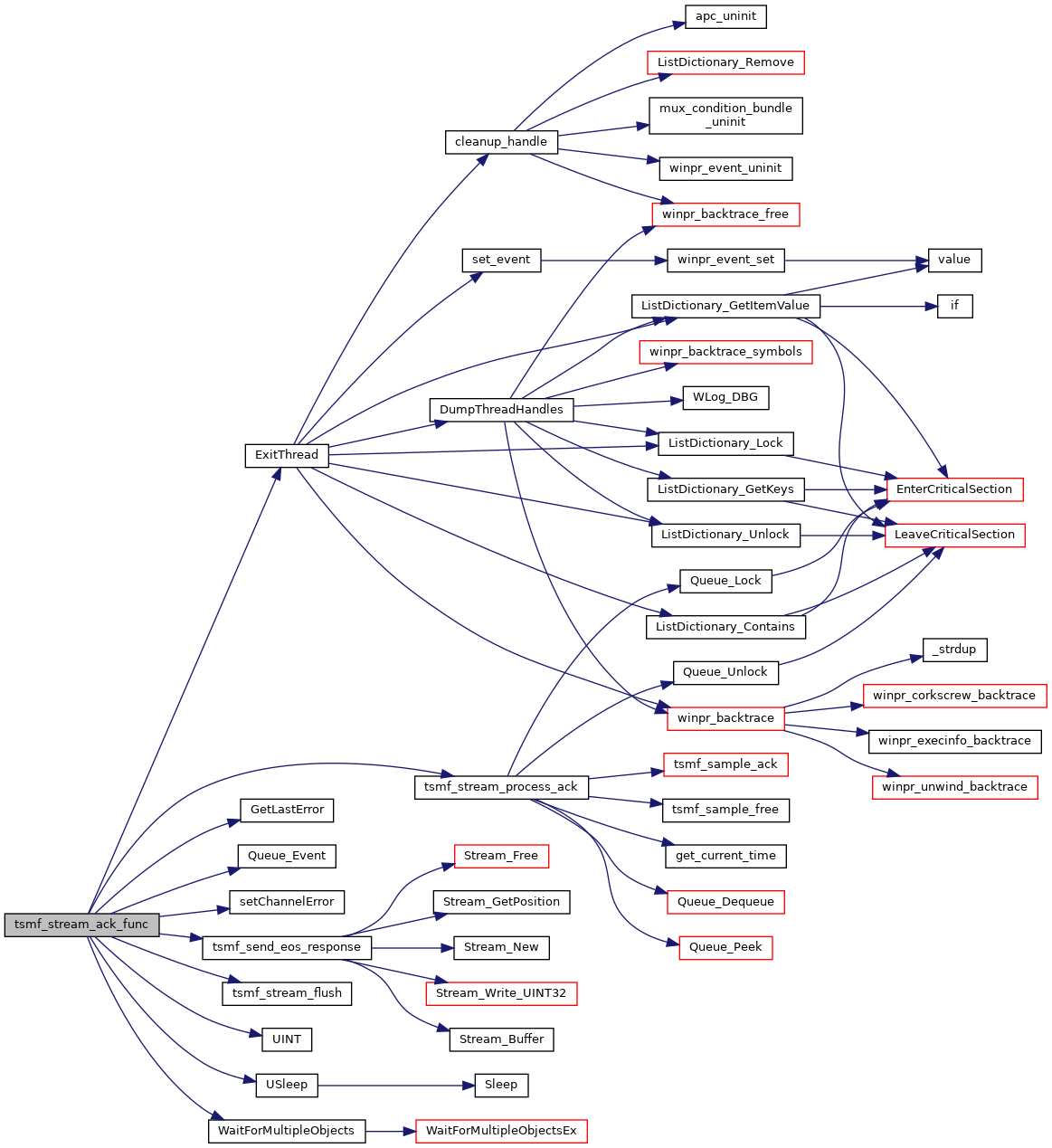Here is the caller graph for this function: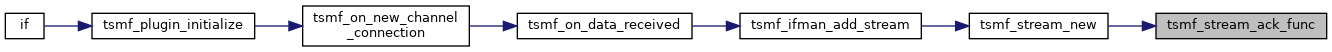## ◆ tsmf_stream_change_volume()

 static BOOL tsmf_stream_change_volume ( TSMF_STREAM * stream, UINT32 newVolume, UINT32 muted )
static
Here is the caller graph for this function: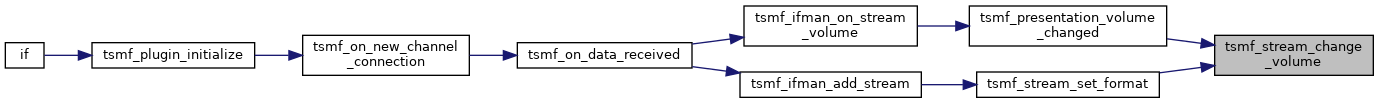## ◆ tsmf_stream_end()

 void tsmf_stream_end ( TSMF_STREAM * stream, UINT32 message_id, IWTSVirtualChannelCallback * pChannelCallback )
Here is the caller graph for this function:## ◆ tsmf_stream_find_by_id()

 TSMF_STREAM* tsmf_stream_find_by_id ( TSMF_PRESENTATION * presentation, UINT32 stream_id )
Here is the call graph for this function:Here is the caller graph for this function: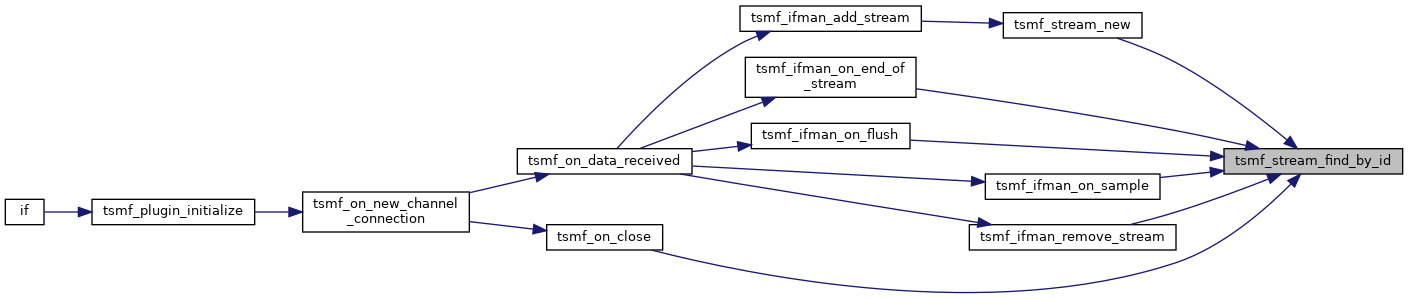## ◆ tsmf_stream_flush()

 BOOL tsmf_stream_flush ( TSMF_STREAM * stream )
Here is the caller graph for this function: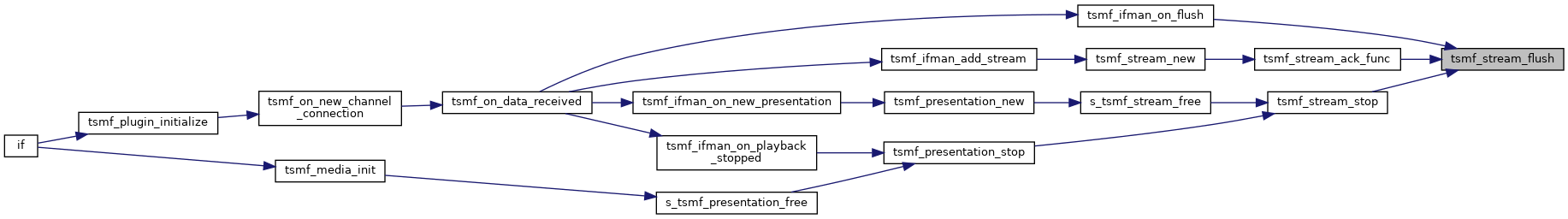## ◆ tsmf_stream_free()

 void tsmf_stream_free ( TSMF_STREAM * stream )
Here is the call graph for this function:Here is the caller graph for this function: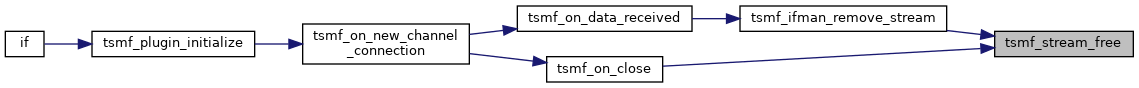## ◆ tsmf_stream_new()

 TSMF_STREAM* tsmf_stream_new ( TSMF_PRESENTATION * presentation, UINT32 stream_id, rdpContext * rdpcontext )
Here is the call graph for this function: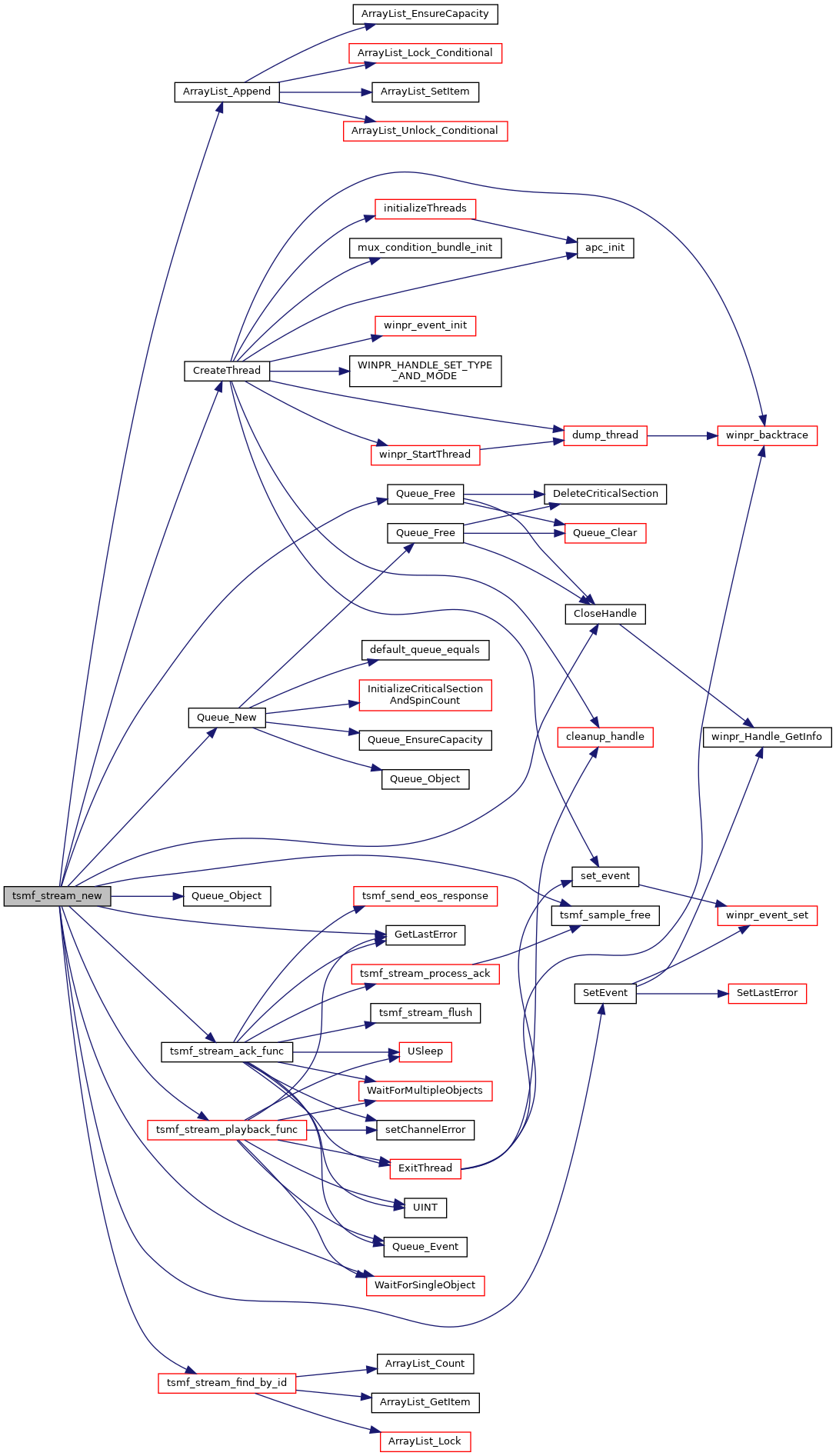Here is the caller graph for this function:## ◆ tsmf_stream_pause()

 static BOOL tsmf_stream_pause ( TSMF_STREAM * stream )
static
Here is the caller graph for this function: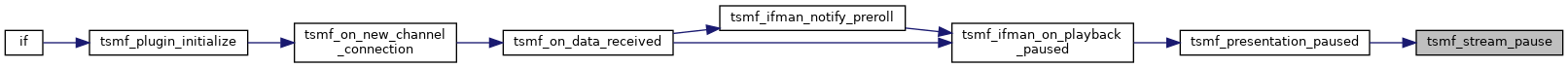## ◆ tsmf_stream_playback_func()

 static DWORD WINAPI tsmf_stream_playback_func ( LPVOID arg )
static
Here is the call graph for this function: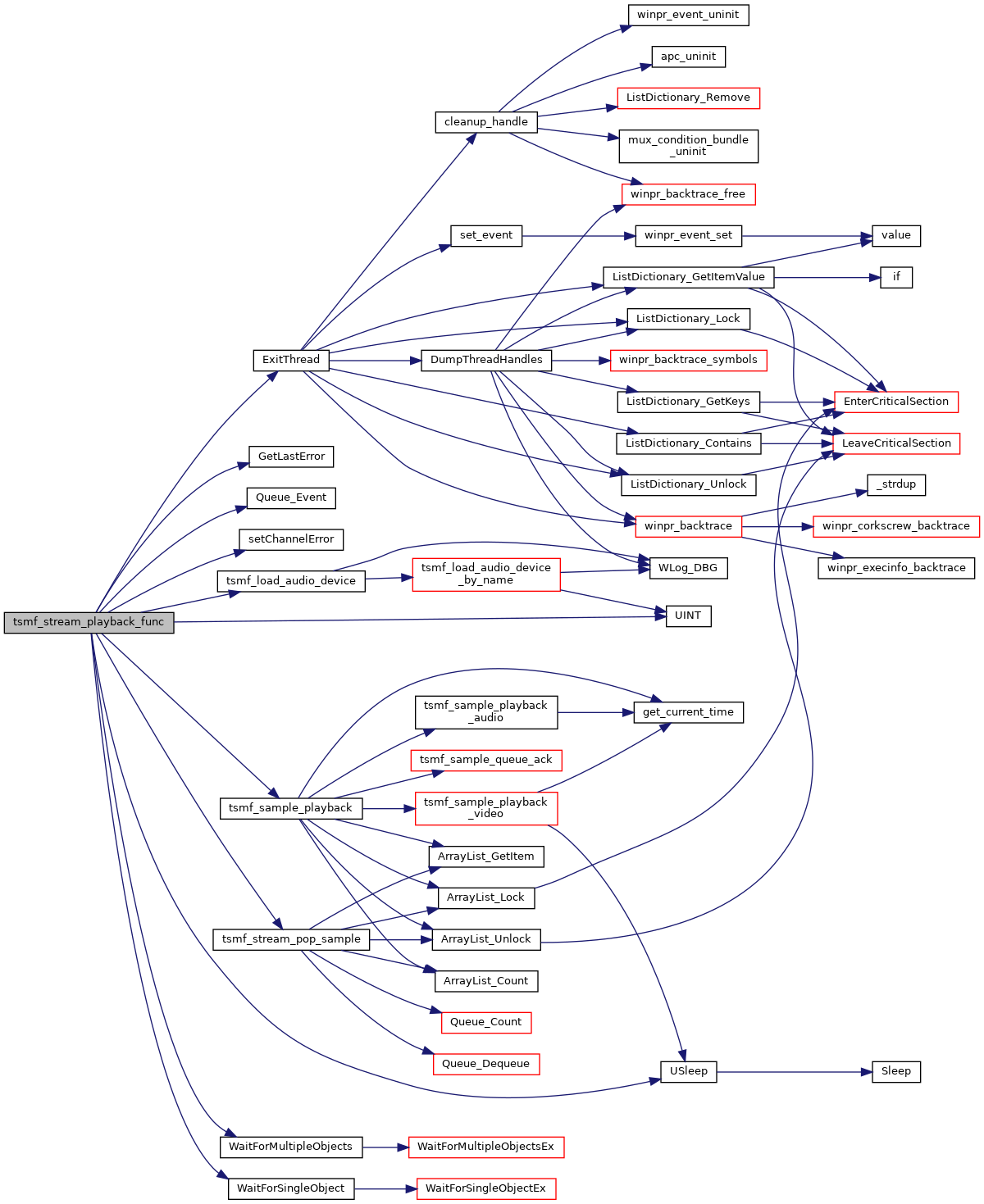Here is the caller graph for this function: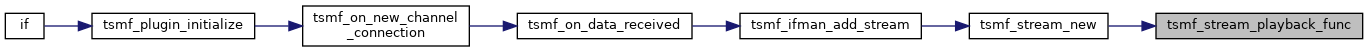## ◆ tsmf_stream_pop_sample()

 static TSMF_SAMPLE* tsmf_stream_pop_sample ( TSMF_STREAM * stream, int sync )
static
Here is the call graph for this function:Here is the caller graph for this function: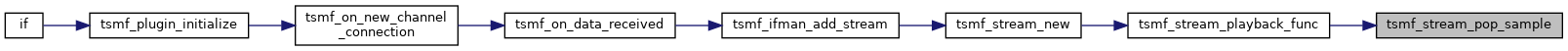## ◆ tsmf_stream_process_ack()

 static BOOL tsmf_stream_process_ack ( void * arg, BOOL force )
static
Here is the call graph for this function:Here is the caller graph for this function: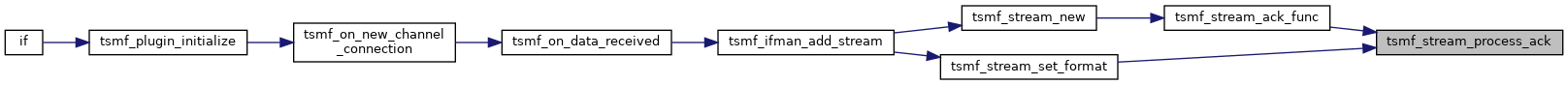## ◆ tsmf_stream_push_sample()

 BOOL tsmf_stream_push_sample ( TSMF_STREAM * stream, IWTSVirtualChannelCallback * pChannelCallback, UINT32 sample_id, UINT64 start_time, UINT64 end_time, UINT64 duration, UINT32 extensions, UINT32 data_size, BYTE * data )
Here is the call graph for this function: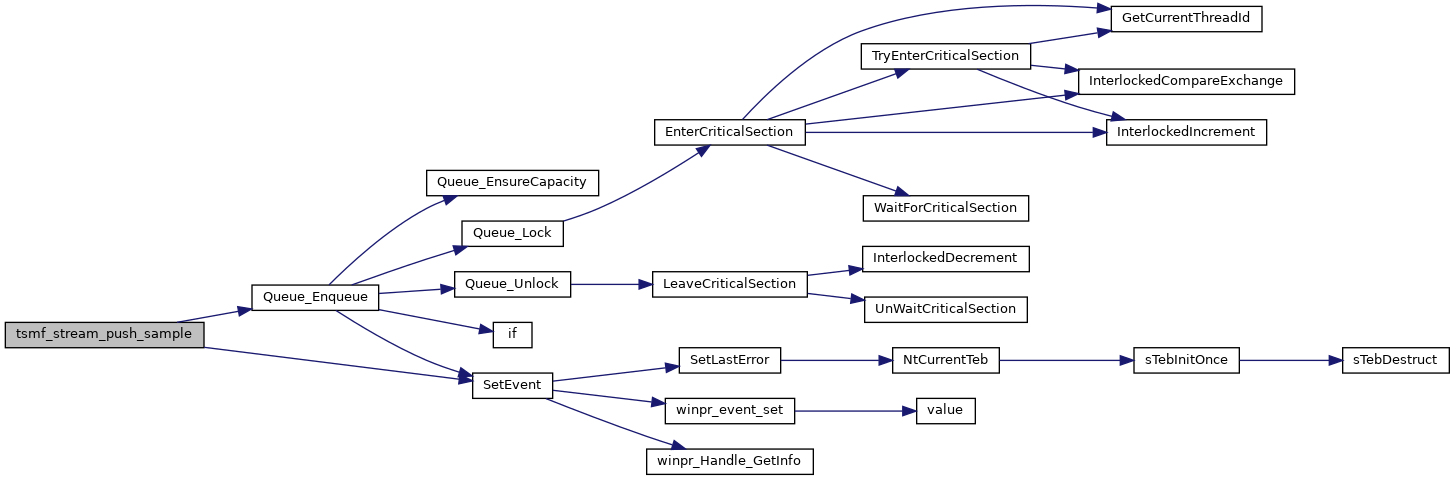Here is the caller graph for this function: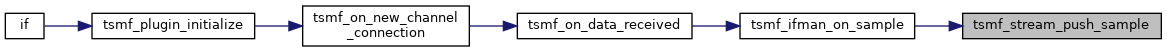## ◆ tsmf_stream_restart()

 static BOOL tsmf_stream_restart ( TSMF_STREAM * stream )
static
Here is the caller graph for this function: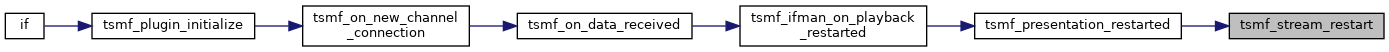## ◆ tsmf_stream_resync()

 static void tsmf_stream_resync ( void * arg )
static
Here is the call graph for this function: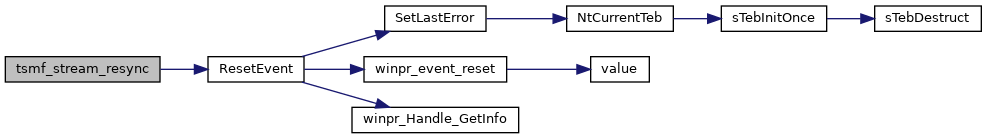Here is the caller graph for this function: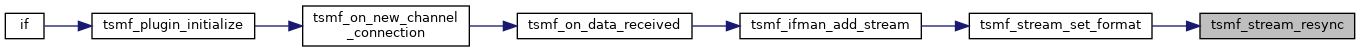## ◆ tsmf_stream_set_format()

 BOOL tsmf_stream_set_format ( TSMF_STREAM * stream, const char * name, wStream * s )
Here is the call graph for this function:Here is the caller graph for this function: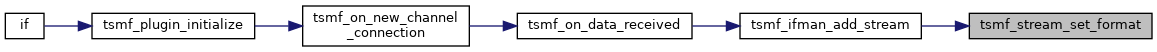## ◆ tsmf_stream_start()

 static BOOL tsmf_stream_start ( TSMF_STREAM * stream )
static
Here is the caller graph for this function: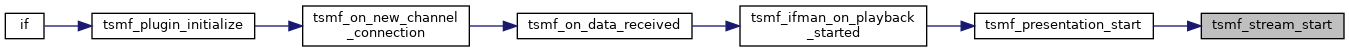void tsmf_stream_start_threads ( TSMF_STREAM * stream )
Here is the call graph for this function: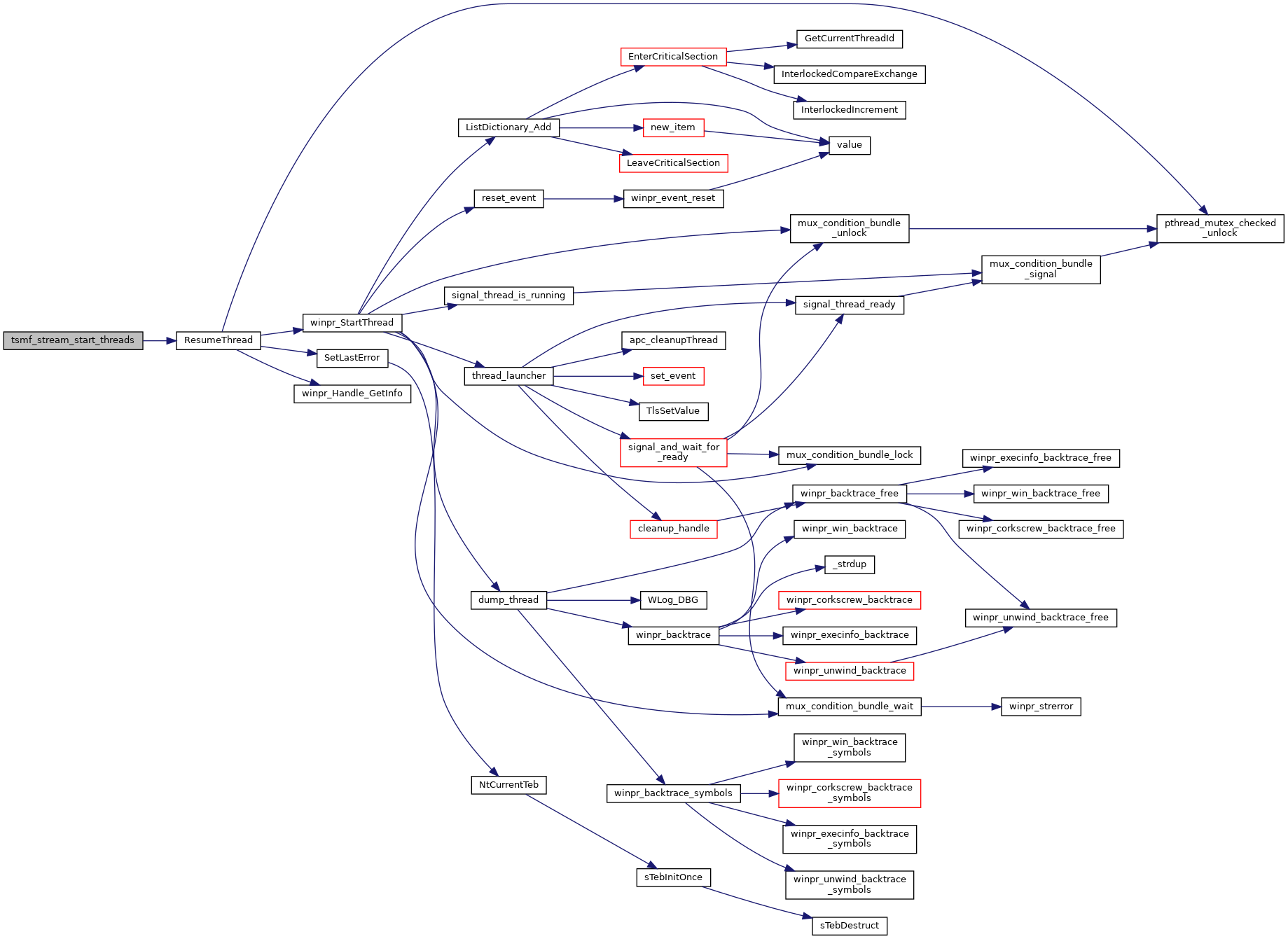Here is the caller graph for this function:## ◆ tsmf_stream_stop()

 static BOOL tsmf_stream_stop ( TSMF_STREAM * stream )
static
Here is the call graph for this function: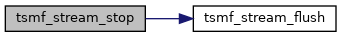Here is the caller graph for this function: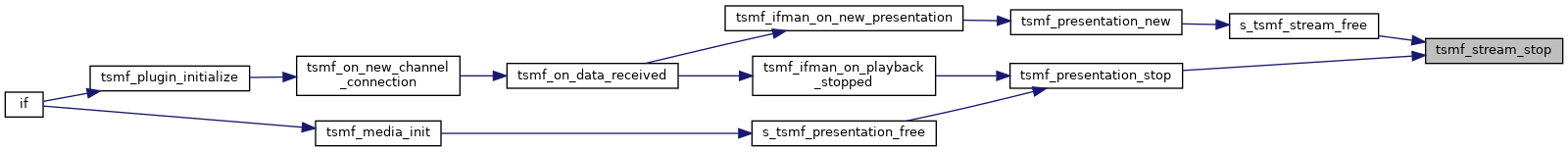## ◆ presentation_list

 wArrayList* presentation_list = NULL
static

## ◆ TERMINATING

 int TERMINATING = 0
static